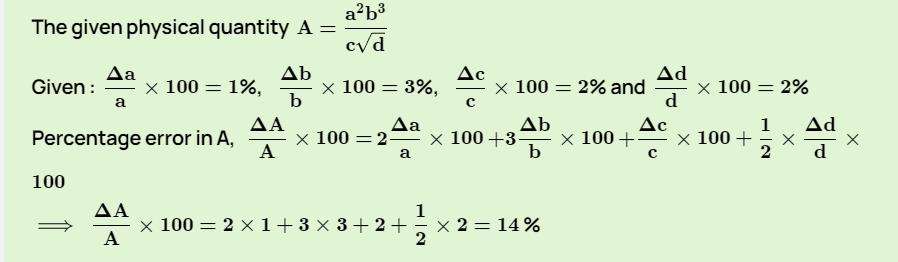Courses

# Test: Measurement & Error Analysis

## 10 Questions MCQ Test Physics For JEE | Test: Measurement & Error Analysis

Description
This mock test of Test: Measurement & Error Analysis for JEE helps you for every JEE entrance exam. This contains 10 Multiple Choice Questions for JEE Test: Measurement & Error Analysis (mcq) to study with solutions a complete question bank. The solved questions answers in this Test: Measurement & Error Analysis quiz give you a good mix of easy questions and tough questions. JEE students definitely take this Test: Measurement & Error Analysis exercise for a better result in the exam. You can find other Test: Measurement & Error Analysis extra questions, long questions & short questions for JEE on EduRev as well by searching above.
QUESTION: 1

### The accuracy in the measurement of time for 20 oscillations of pendulum for the following measurements, t1 = 39.6 s, t2 = 39.9 s and t3 = 39.5 s is:

Solution:

According to the problem time for 20 oscillations of a pendulum

t​= 3 9.6s,  t​= 39.9s,  t3​ =3 9.5s

It is quite obvious from these observations that the least count by the water is 0.1s. As measurements have only one decimal place.

► Precision in measurement = least count of measuring instrument = 0.1s

► Precision in 20 oscillations = 0.1

Therefore, Precision in 1 oscillation = 0.1/ 20​= 0.005.

QUESTION: 2

### Which of the following is caused by careless handling?

Solution:

Gross errors are mostly due to lack of knowledge, judgment and care on the part of experiment. That is Gross error is caused by careless handling.

QUESTION: 3

### A student measures the distance covered and time taken by a body under free fall which was initially at rest. He uses this data for estimating g, the acceleration due to gravity. If the maximum percentage error in measurement  of the distance and the time are e1 and e2 respectively, then the maximum possible percentage error in the estimation of g is:

Solution:

From the releation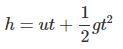h= 1/2gt ⇒ g = 2h/t2

Taking natural logarithms on both sides, we get

ln (g)  = ln (h) - 2ln (t) + ln (2)

Differentiating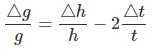for maximum permissible errors , we add both the errors . so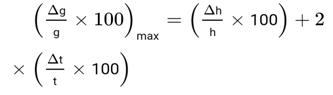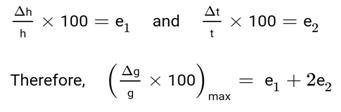QUESTION: 4

Error in the measurement of radius of a sphere is 1%. The error in the calculated value of its volume is:

Solution:

∵ V = (4/3)πr3
% error is volume = 3 × error in radius = 3 × 1 = 3%

QUESTION: 5

The error in two readings of A are 0.01 and -0.03, then mean absolute error is?

Solution:

Absolute error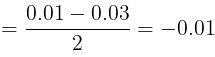Mean absolute error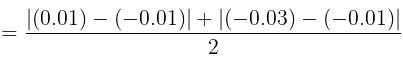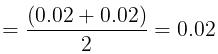QUESTION: 6

At a certain place, the acceleration due to gravity is determined using a simple pendulum. The length of the pendulum is 25.0 cm and a stopwatch with least count is 1 s measures the time taken by the pendulum for 40 oscilations to be 50 s. the accuracy in the measurement of g is:

Solution: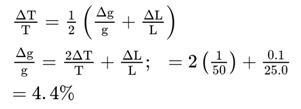QUESTION: 7

The most suitable instrument for measuring the size of an atom is:

Solution:

Only electron microscope has resolution of 0.5-0.6 Angstrom which is less than the size of an atom.

QUESTION: 8

Which is the most accurate atomic clock?

Solution:

Cesium 133 is the element most commonly chosen for atomic clocks.

QUESTION: 9

The method used to measure the distance of a planet from the earth is:

Solution:

The astronomers mainly use parallax method to find distances to objects like other planets. To calculate the distance to a star or to a planet, astronomers observe it from different places along Earth's orbit around the Sun.

QUESTION: 10

What is the percentage error in the physical quantity A if it is related to four other physical quantities a, b, c and d as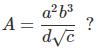The percentage error in measurement of a, b, c and d are 1%, 3%, 2% and 2% respectively.

Solution: